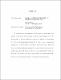## Moduli Spaces of Sheaves on Hirzebruch OrbifoldsWe provide a stacky fan description of the total space of certain split vector bundles, as well as their projectivization, over toric Deligne-Mumford stacks. We then specialize to the case of Hirzebruch orbifold $\mathcal{H}_{r}^{ab}$ obtained by projectivizing $\mathcal{O} \oplus \mathcal{O}(r)$ over the weighted projective line $\mathbb{P}(a,b)$. Next, we give a combinatorial description of toric sheaves on $\mathcal{H}_{r}^{ab}$ and investigate their basic properties. With fixed choice of polarization and a generating sheaf, we describe the fixed point locus of the moduli scheme of $\mu$-stable torsion free sheaves of rank $1$ and $2$ on $\mathcal{H}_{r}^{ab}$. Finally, we show that if $\mathcal{X}$ is the total space of the canonical bundle over a Hirzebruch orbifold, then we can obtain generating functions of Donaldson-Thomas invariants.